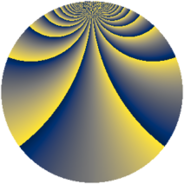Properties

 Label 588.2.baLevel $588$ Weight $2$ Character orbit 588.ba Rep. character $\chi_{588}(103,\cdot)$ Character field $\Q(\zeta_{42})$ Dimension $672$ Newform subspaces $2$ Sturm bound $224$ Trace bound $3$

Related objects

Defining parameters

 Level: $$N$$ $$=$$ $$588 = 2^{2} \cdot 3 \cdot 7^{2}$$ Weight: $$k$$ $$=$$ $$2$$ Character orbit: $$[\chi]$$ $$=$$ 588.ba (of order $$42$$ and degree $$12$$) Character conductor: $$\operatorname{cond}(\chi)$$ $$=$$ $$196$$ Character field: $$\Q(\zeta_{42})$$ Newform subspaces: $$2$$ Sturm bound: $$224$$ Trace bound: $$3$$ Distinguishing $$T_p$$: $$11$$

Dimensions

The following table gives the dimensions of various subspaces of $$M_{2}(588, [\chi])$$.

Total New Old
Modular forms 1392 672 720
Cusp forms 1296 672 624
Eisenstein series 96 0 96

Trace form

 $$672 q - 12 q^{8} + 56 q^{9} + O(q^{10})$$ $$672 q - 12 q^{8} + 56 q^{9} + 18 q^{10} + 22 q^{14} + 8 q^{16} + 8 q^{21} + 12 q^{22} + 24 q^{24} - 52 q^{25} - 30 q^{26} + 36 q^{28} - 8 q^{30} - 10 q^{32} + 12 q^{33} + 112 q^{34} + 32 q^{37} - 18 q^{38} - 26 q^{40} - 16 q^{42} - 64 q^{44} - 8 q^{49} - 228 q^{50} - 36 q^{52} - 8 q^{53} - 40 q^{56} - 24 q^{57} - 6 q^{58} + 10 q^{60} + 4 q^{61} - 8 q^{65} + 36 q^{66} + 36 q^{68} - 108 q^{70} + 48 q^{72} + 36 q^{73} + 22 q^{74} + 42 q^{76} - 16 q^{77} - 12 q^{78} - 54 q^{80} + 56 q^{81} - 186 q^{82} + 40 q^{84} + 48 q^{85} - 188 q^{86} - 284 q^{88} + 28 q^{92} - 4 q^{93} - 336 q^{94} - 30 q^{96} - 278 q^{98} + O(q^{100})$$

Decomposition of $$S_{2}^{\mathrm{new}}(588, [\chi])$$ into newform subspaces

Label Dim. $$A$$ Field CM Traces $q$-expansion
$a_{2}$ $a_{3}$ $a_{5}$ $a_{7}$
588.2.ba.a $336$ $4.695$ None $$0$$ $$-28$$ $$0$$ $$2$$
588.2.ba.b $336$ $4.695$ None $$0$$ $$28$$ $$0$$ $$-2$$

Decomposition of $$S_{2}^{\mathrm{old}}(588, [\chi])$$ into lower level spaces

$$S_{2}^{\mathrm{old}}(588, [\chi]) \cong$$ $$S_{2}^{\mathrm{new}}(196, [\chi])$$$$^{\oplus 2}$$